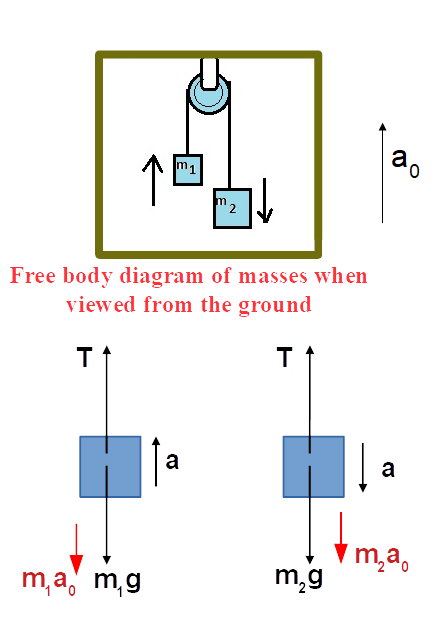# Masses m_1and m_2 are connected by a light string running over a frictionless pulley attached to...

## Question:

Masses {eq}m_1 {/eq} and {eq}m_2 {/eq} are connected by a light string running over a frictionless pulley attached to an elevator. The elevator starts to move up with acceleration. Find tension force on {eq}m_1 {/eq} and {eq}m_2 {/eq} with respect to ground.

## Pseudo Force:

When the motion of an object is described using a non-inertial reference frame (a frame having non-zero acceleration) then, an apparent force acts on the object. This apparent force is known as Pseudo force.

It is also called a fictitious force, inertial force or d'Alembert force.

Given:

• Block 1 has mass {eq}m_1 {/eq} and block 2 has mass {eq}m_2 {/eq}

Let

• {eq}a_0 {/eq} is the acceleration of the elevator along upward direction.

The free-body diagram of blocks is shown in the figure below.In the above diagram,

• {eq}T {/eq} is the tension in the string.
• {eq}a {/eq} is the acceleration of the blocks with respect to the elevator.
• {eq}m_1a_0 {/eq} is the pseudo force on the block 1 when observed by an observer sitting on the elevator.
• {eq}m_2a_0 {/eq} is the pseudo force on the block 2 when observed by an observer sitting on the elevator.

Applying law of motion along vertical direction for the blocks.

{eq}T -m_1g-m_1a_0 = m_1a \ \ \ \ \ \ \ \ \ \ \ \ \ \ \ \ \ \ \ \ \ \ \ \ \ \ \ \ \ \ \ \ \ \ Eqn.(1) {/eq}

{eq}m_2g+m_2a_0-T = m_2a \ \ \ \ \ \ \ \ \ \ \ \ \ \ \ \ \ \ \ \ \ \ \ \ \ \ \ \ \ \ \ \ \ \ Eqn.(2) {/eq}

From equation 1 and 2,

{eq}\begin{align} a &=\dfrac{(m_2-m_1)(g+a_0)}{(m_1+m_2)}\\ \end{align} {/eq}

and

{eq}\begin{align} T &=m_1a+m_1g+m_1a_0 \\ &=m_1 \left \{ \dfrac{(m_2-m_1)(g+a_0)}{(m_1+m_2)}+(g+a_0) \right \}\\ &= \dfrac{2m_1m_2(g+a_0)}{(m_1+m_2)} \\ \end{align} {/eq}

Therefore, the tension force on {eq}m_1 {/eq} and {eq}m_2 {/eq} is {eq}T = \dfrac{2m_1m_2(g+a_0)}{(m_1+m_2)} {/eq}